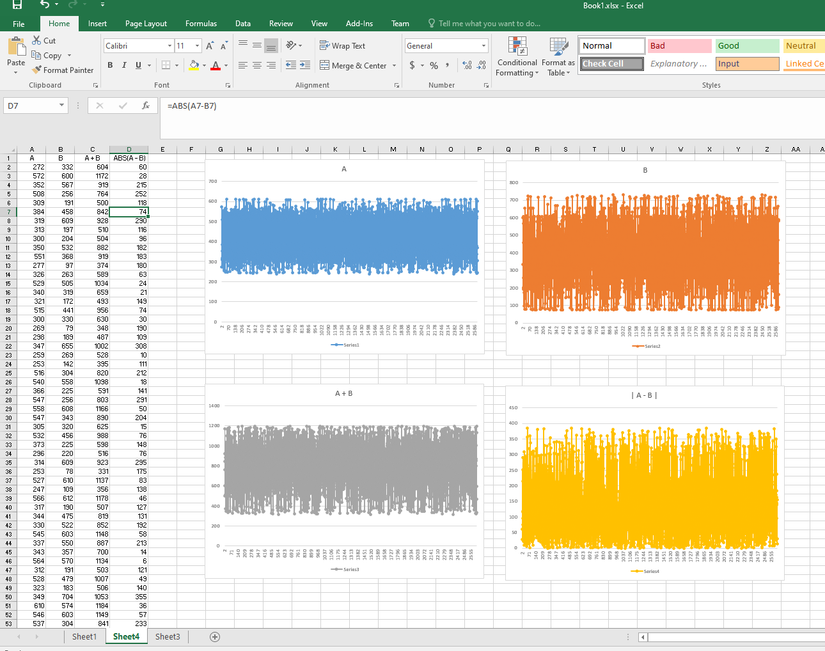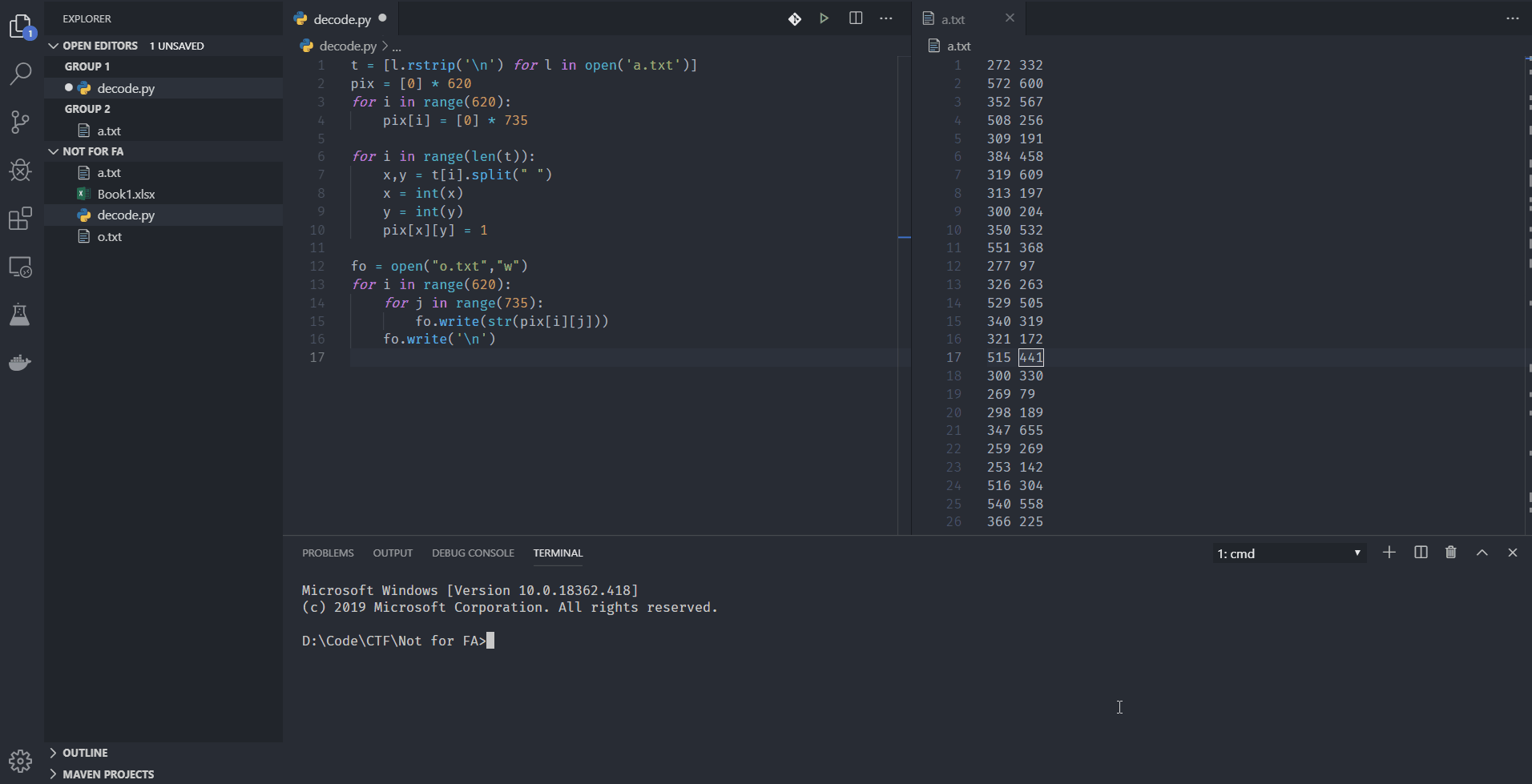[Write-up] Not for FA

Prompt: Together, those couples will tell the flag :v https://controlc.com/8b1286d4

Overview

At first, we are presented with a list of 2617 pairs of numbers. Let's call the first column A, and the other, B.

• 2617 is a prime number so this sequence of numbers most likely unrelated to any "base" encoding
• A list of 2617 characters is roughly the length of a paragraph => this could not be the flag (but may contain the flag so we will try to decode it anyway)
• The prompt emphasizes together & those couples. Therefore, there is a strong link between A and B
A | B
...
272 332
572 600
352 567
508 256
309 191
384 458
319 609
313 197
300 204
...

Digging deep down - First approach

We try to find the strong connection mentioned above, so I fired up Excel:Blue Graph Orange Graph Grey Graph Yellow Graph
Represent A B A + B |A - B|
Max 610 732 1196 385
Min 237 74 316 0

From the table, we can come to our first points:
• The numers are not related to ASCII (range 237-610 and 74-732)
• The sums and differences between A and B are too meaningless (I thought that |A-B| will output ASCII numbers)

Sidenote: I also tried to XOR, AND, and OR those numbers but nothing poped up. Also, it's 3AM already, I should take a break.

Another mindset - Second approach

The day after, I carefully read the prompt again

Together, those couples will tell the flag

Hang on, these numbers come in pairs, and so do the cordinates. Maybe the A and B are actually X and Y on the plane/bitmap.

Using data from the table above, we can determine the height and width of bitmap.

X Y
Max 610 732

I wrote a python script to parse the values:
t = [l.rstrip('\n') for l in open('a.txt')]
pix =  * 620
for i in range(620):
pix[i] =  * 735

for i in range(len(t)):
x,y = t[i].split(" ")
x = int(x)
y = int(y)
pix[x][y] = 1

fo = open("o.txt","w")
for i in range(620):
for j in range(735):
fo.write(str(pix[i][j]))
fo.write('\n')

The flag is in o.txtEnjoy CTF!

Written by dnhuan (fb.com/dnhuan)